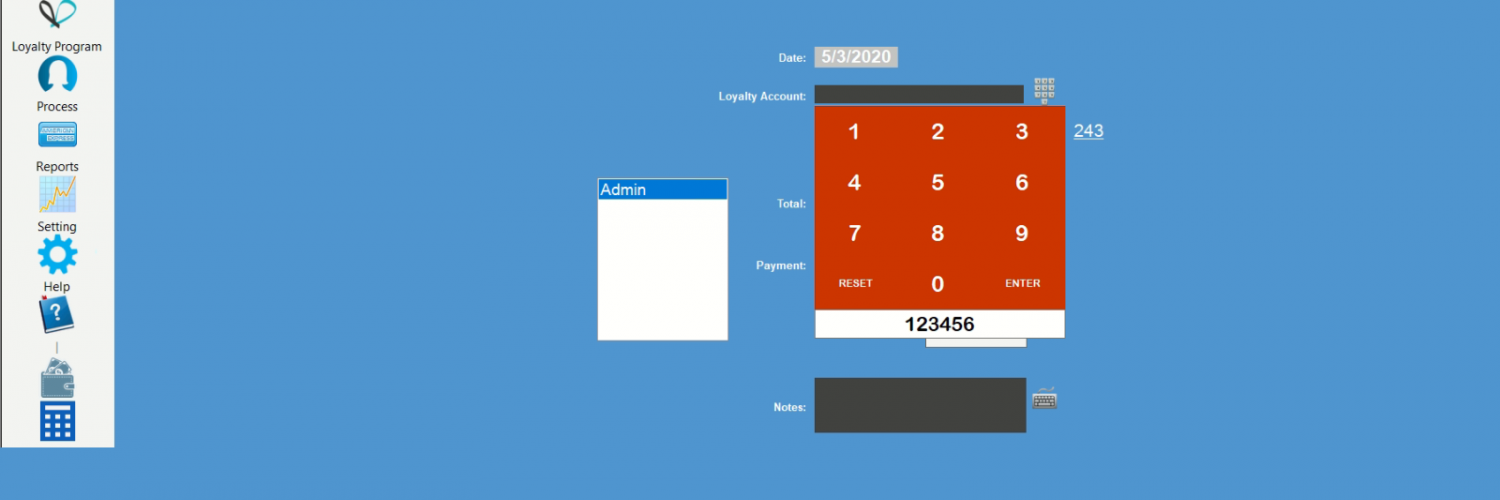# Automatically format payment amount entry in C#

## Introduction

For end-users, it is much easier if they could enter the digits from a numeric keypad, and the currency format automatically applies, instead of entering a decimal point and numbers!

## Background

In many PoS software applications (or similar environments), a payment screen requires a “payment amount entry.” Payment values (currency) almost always include a decimal point. So, if we don’t handle the decimal point and leave it to the user, it could (it will !) become a point of failure. When the user enters more than one decimal point or adds the decimal point where it changes the total amount (A \$10.25 payments becomes \$102.50) So, you either have to check the value or catch it at keypress level. A better method is to manage it at the entry point!

## Using the code

On a Windows form, add buttons and build your “Price numeric keypad” . I have used a TableLayoutPanel of 4×4. Add a Textbox under the keypad grid, for the output.

I have introduced a public string, which decides what role our keypad will be playing:

``public string numberRole = "MONEY" ;``

“Money” role will set the stage for a payment value where a two digits decimal will be applied. For instance, if the amount is 10.25, we will have the followings:

step 1: 0.01

step 2: 0.10

step 3: 1.02

step 4: 10.25

“DIGITS” role will turn our keypad into a numeric, without the decimal point.

I also control the number of decimal points and also decimal digits(default is 2).

MakeAmount is the critical player in this code.

``````private void MakeAmount(string amountTxt)
{
decimal meanWhile;
txtValue.Text = txtValue.Text + amountTxt;
if (txtValue.Text.Length == 1)
{
txtValue.Text = "0.0" + txtValue.Text;
}
if (txtValue.Text.Length > 4)
{
meanWhile = Convert.ToDecimal(txtValue.Text) * 10;
txtValue.Text = meanWhile.ToString("G29");
}
}``````

As you can see, it depends on what we have; the decimal points get moved.
For every digit, we have the same process, Except for zero, which we need to check a few things, all other Click events are similar:

``````private void num2_Click(object sender, EventArgs e)
{
if (numberRole == "DIGITS")
{
txtValue.Text = txtValue.Text + "2" ;
} else MakeAmount(num2.Text);
}``````

And for zero:

``````private void num0_Click(object sender, EventArgs e)
{
decimal meanWhile;
if (numberRole == "DIGITS")
{
txtValue.Text = txtValue.Text + "0";
}
else
{
txtValue.Text = txtValue.Text + "0";
if (txtValue.Text.Length == 1)
{
txtValue.Text = "0.0" + txtValue.Text;
}
if (txtValue.Text.Length >= 4)
{
meanWhile = Convert.ToDecimal(txtValue.Text) * 10;
txtValue.Text = meanWhile.ToString("G29");
}
if (Convert.ToDecimal(txtValue.Text) < 1)
{
txtValue.Text = txtValue.Text + "0";
}
if (txtValue.Text.IndexOf(".") < 0)
{
txtValue.Text = txtValue.Text + ".00";
}
if (Convert.ToDecimal(txtValue.Text) > 1 && (txtValue.Text.Length - txtValue.Text.IndexOf(".") - 1 == 1))
{
txtValue.Text = txtValue.Text + "0";
}
}
}``````

``````numberpad showNumber = new numberpad();
showNumber.numberRole = "MONEY";``````

As you can see, this calls for a “Money” format.
And if you need a numeric keypad, call your function with “DIGITS”:

``````numberpad showNumber = new numberpad();
showNumber.numberRole = "DIGITS";``````